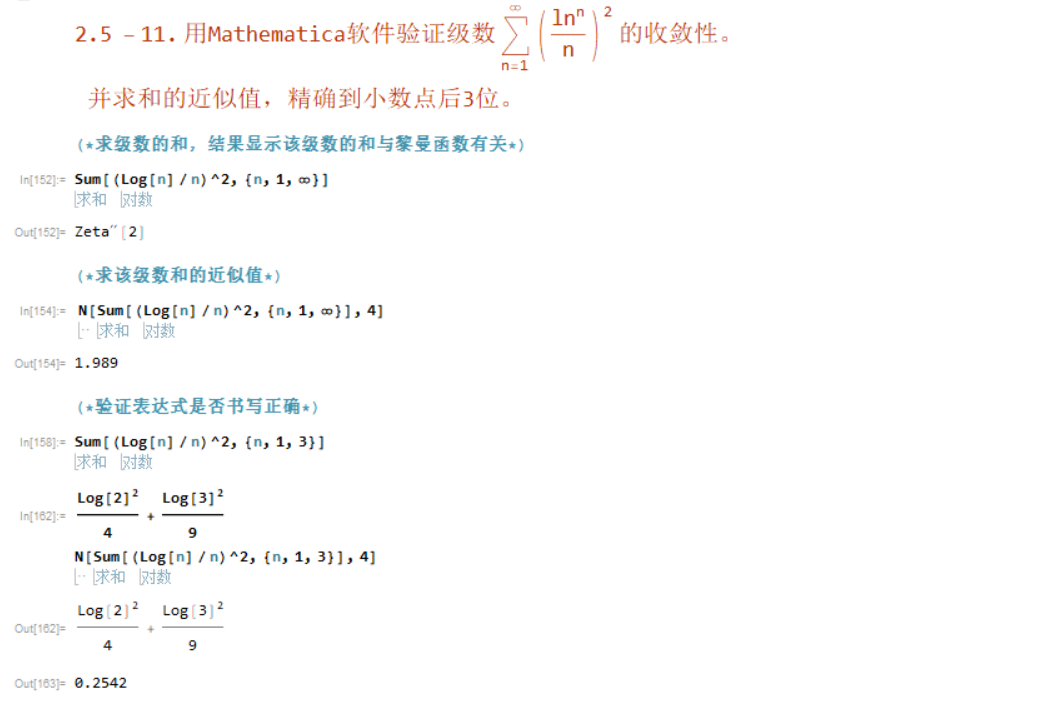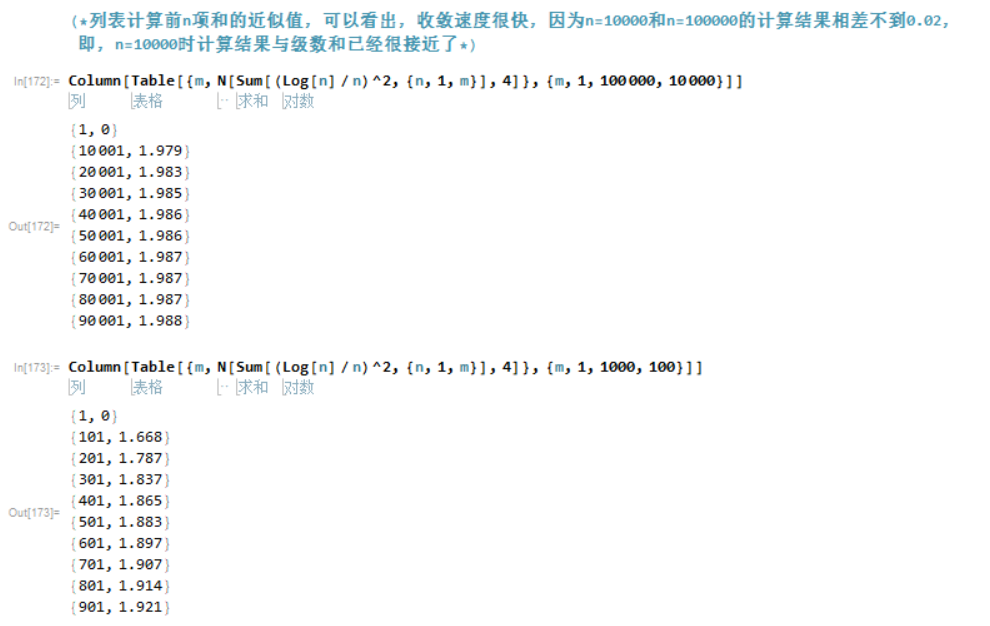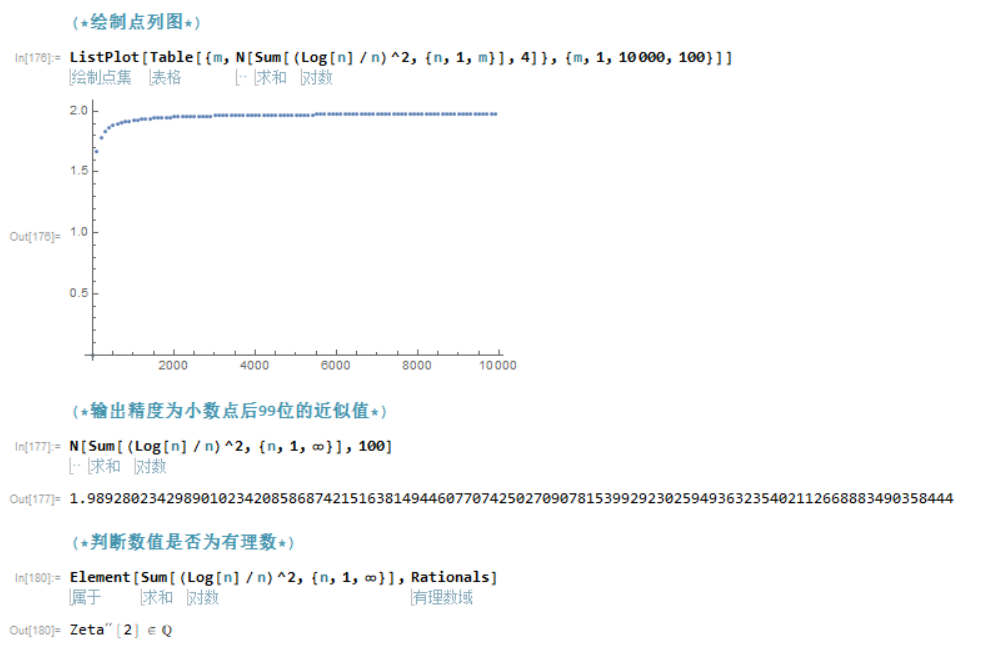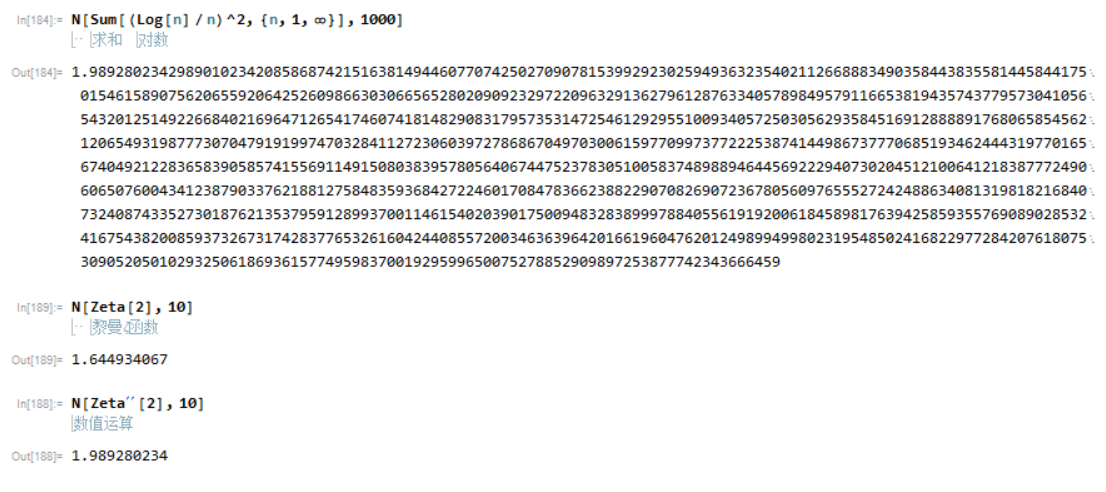# 高等数学Mathematica实验题——2.5 - 11. 验证级数的收敛性

2.5 -11. 用Mathematica软件验证级数 \!$$\*UnderoverscriptBox[\(\[Sum]$$, $$n = 1$$, $$\[Infinity]$$]$$\*SuperscriptBox[\(( \*FractionBox[ SuperscriptBox[\(ln$$, $$n$$], $$n$$])\), $$2$$]\ 的收敛性\)\)。并求和的近似值，精确到小数点后3位。